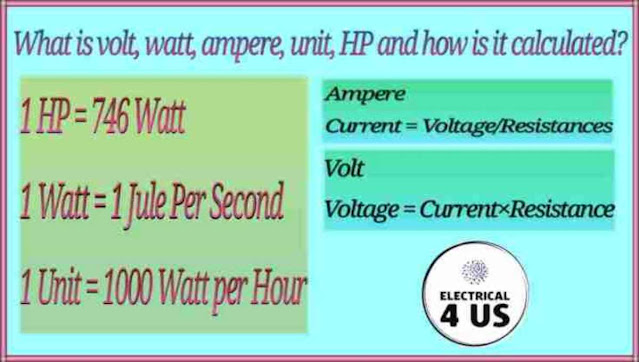## Posts

Showing posts with the label Ampear

### What is ampere, volt, hp, watt and unit?Many things happen in electricity. About whom we must have knowledge. What is volt, watt, ampere, unit, HP and how is it calculated? What is Ampere? The unit of electric current is ampere A. According to Ohm's law Current = Voltage / Resistance What is a volt? EMF means the unit of electric carrying force is volt. If 1 ampere to 1 ampere current is given, then it is called one volt. According to Ohm's law Voltage = Current × Resistance What is HP? HP means: Horse Power 1 British HP = 746 watts 1 Metric HP = 735.5 Watt What is watt? The unit of electric power is watts 1 watt = 1 joule per second Power = Voltage × Current What is a unit? The unit of electrical energy is the unit. 1 Unit = 1 kilowatt per hour Or 1 unit = 1000 watt per hour